×

Search anything:

# Techniques to detect Deepfake videos

#### Deep Learning Machine Learning (ML)Get this book -> Problems on Array: For Interviews and Competitive Programming

Hello everyone, Today we are going to discuss one of the most morally sensitive subject in Artificial Intelligence world which are DeepFake and how we try to detect the deep fake from real.

1. Deepfake and its Generation.
2. Problems of Deepfakes.
3. Deepfake Detection using MesoNet-4.
4. Datasets used.
5. Other techniques.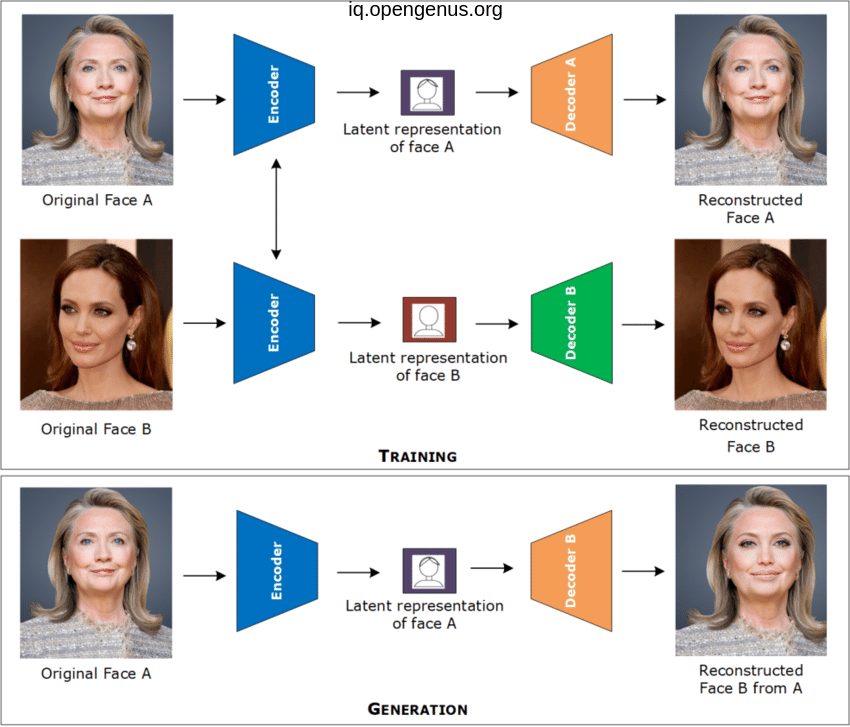.

### 1. Deepfake and its Generation :

We can define tge deepfake as realistic-looking falsified images and videos created by AI algorithms specially after the strong development of deep learning technology such as GANs and Auto-encoder architectures.

Mainly Auto-encoder architecture or GANs is used to generate deepfake images or videos.

The encoder compress the image to get rid of noise then the decoder will restore the image or highly approximate to it.

But if we compress an image using a general encoder then pass the compressed image to another decoder for another image it will lead to another style of image or a deep fake image.

Also GANs can generate a deepfake images as the generator receives random inputs seeds to generate a fake sample. Those fake samples are used to train the discriminator. The discriminator is simply a binary classifier, and it takes the real samples and fake samples as inputs and then, discriminator applies a SoftMax function to distinguish the realistic data from the fake one.

CycleGAN and VGGFace are a GAN based algorithms for deepfake generation.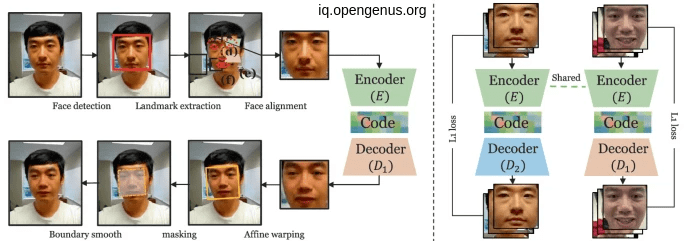.

### 2. Problems of Deepfakes:

Although first deepfake techniques was invented for lowering cost of video campaigns and give a high personalized customers experience But their risks and hazards make it a must to find an anti-deepfake techniques, and you may wounder what's the problems of deepfake ?!!

First of all Identity theft, nowadays we can't even trust the videos ?!!!
creating fake news, false pornographic videos and malicious hoaxes, usually targeting well-known people such as politicians and celebrities. Potentially, deepfakes can be used as a tool for identity theft, extortion, sexual exploitation, reputational damage, ridicule, intimidation and harassment. According to the australian government.

obviously it is a double-edge weapon

### 3. Deepfake Detection:

Through many years there were a struggle between deepfake generation and how to detect this deepfake and one of the most powerful technique for this detection is using MesoNet based on the Inception module the proposed MesoNet, is mainly composed of 4 consecutive convolution layers and two fully connected layers.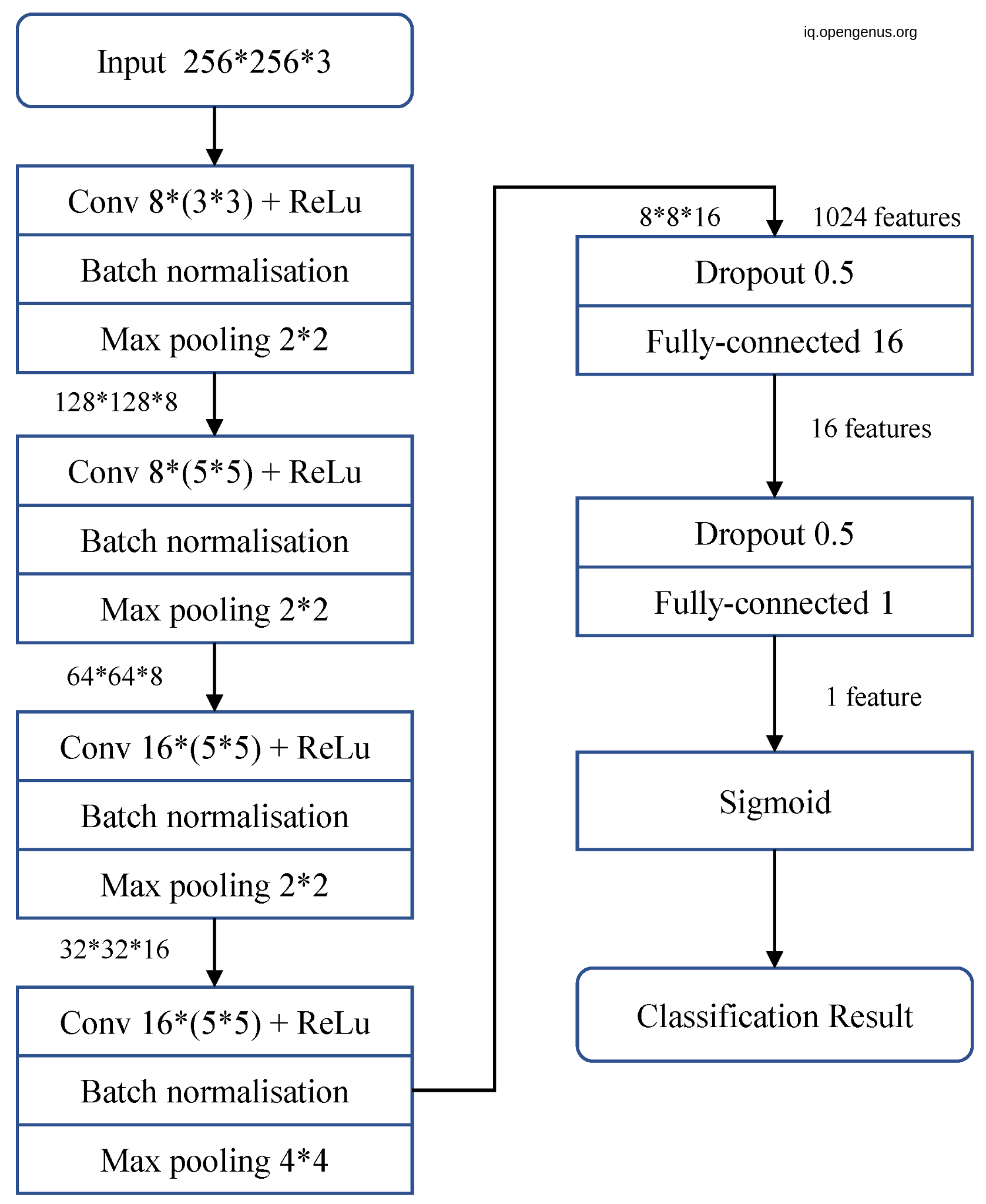.

AND here we are going to dive into the code suggested by the author :
First let's import necessary libraries

``````import numpy as np
import matplotlib.pyplot as plt
from tensorflow.keras.layers import Input, Dense, Flatten, Conv2D, MaxPooling2D, BatchNormalization, Dropout, Reshape, Concatenate, LeakyReLU
from tensorflow.keras.preprocessing.image import ImageDataGenerator
from tensorflow.keras.models import Model
``````

Then save the image dimensions in a dict :

``````image_dimensions = {'height':256, 'width':256, 'channels':3}
``````

Then use OOP to create a classifier class and some methods of it:

``````class Classifier:
def __init__():
self.model = 0

def predict(self, x):
return self.model.predict(x)

def fit(self, x, y):
return self.model.train_on_batch(x, y)

def get_accuracy(self, x, y):
return self.model.test_on_batch(x, y)

``````

then inherit from it and create the mesonet model class:

``````class Meso4(Classifier):
def __init__(self, learning_rate = 0.001):
self.model = self.init_model()
self.model.compile(optimizer = optimizer,
loss = 'mean_squared_error',
metrics = ['accuracy'])

def init_model(self):
x = Input(shape = (image_dimensions['height'],
image_dimensions['width'],
image_dimensions['channels']))

x1 = Conv2D(8, (3, 3), padding='same', activation = 'relu')(x)
x1 = BatchNormalization()(x1)

x2 = Conv2D(8, (5, 5), padding='same', activation = 'relu')(x1)
x2 = BatchNormalization()(x2)

x3 = Conv2D(16, (5, 5), padding='same', activation = 'relu')(x2)
x3 = BatchNormalization()(x3)

x4 = Conv2D(16, (5, 5), padding='same', activation = 'relu')(x3)
x4 = BatchNormalization()(x4)

y = Flatten()(x4)
y = Dropout(0.5)(y)
y = Dense(16)(y)
y = LeakyReLU(alpha=0.1)(y)
y = Dropout(0.5)(y)
y = Dense(1, activation = 'sigmoid')(y)

return Model(inputs = x, outputs = y)

``````

then trying to instantiate a MesoNet model with pretrained weights

``````mesonet = Meso4()
``````

NOW we had a pre-trained model that is capable to differentiate between fake and real image and videos efficiently.

## 4. Datasets used:

We use two public datasets, FF++ and Celeb-DF. the FF++ contain 1000 original videos containing only a single face. and the Celeb-DF considered the second generation of deepfake videos datasets which aim to solve the problem of low-quality generated faces containing 590 original videos and 5639 corresponding DeepFake videos.
there are other many deepfake datasets such as :

• UADFV that contain 49 real videos from youtube that use to create corresponding 49 deep fake ones
• DeepfakeTIMIT 640 deepfake videos are created
• DFD Google deepfake detection datasets that contain 3068 DeepFake videos generated based on 363 original videos.
• DFFD containing 3000 videos that have been manipulated.

## 5. Other techniques

There are other techniques used to detected deepfake videos such as :

• Using Convolutional Vision Transformer: the model consists of two components:the preprocessing component and the detection component. The preprocessing component consists of the face extraction and data augmentation. The
detection components consist of the training component, the validation component, and the testing component.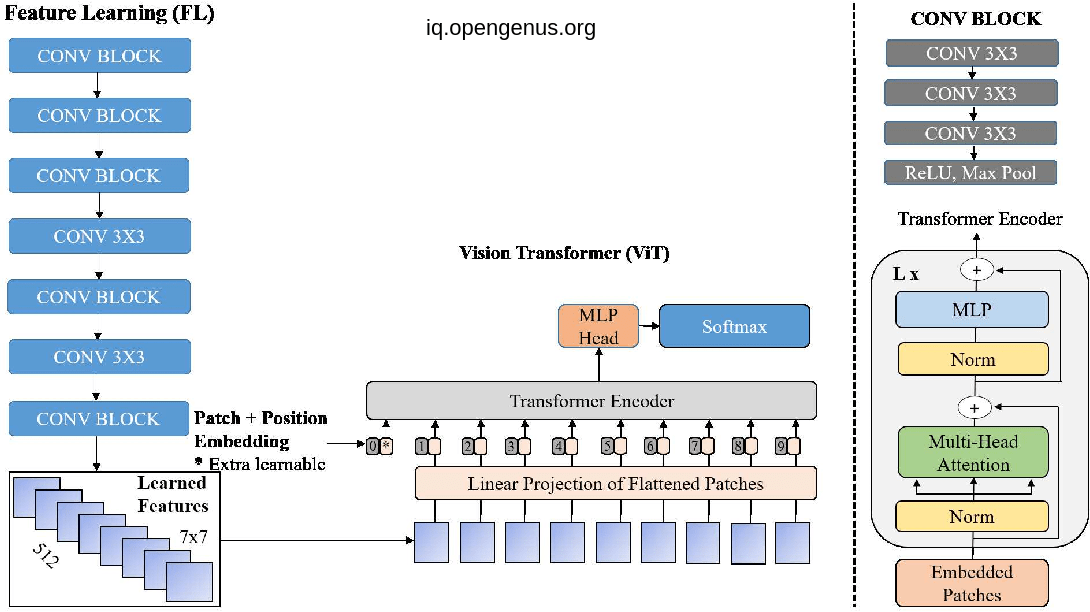• Using a convolutional neural network (CNN) with a recursive neural network (RNN) to discover the physiological signals such eye movement and blinking. Then, the model uses a binary classifier to detect the close and open eyes state.

• Using CNN and LSTM model for analysis a temporal sequence for face manipulation between frames. Finally, a softmax function is used to classify the video as either real or fake.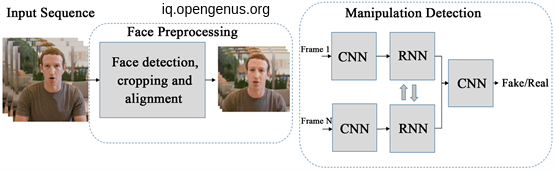Finally I want to thank you for reading.#### Ahmed Mandour

Ahmed Mandour is working at National Food Safety Authority and was studied at Mansoura University.